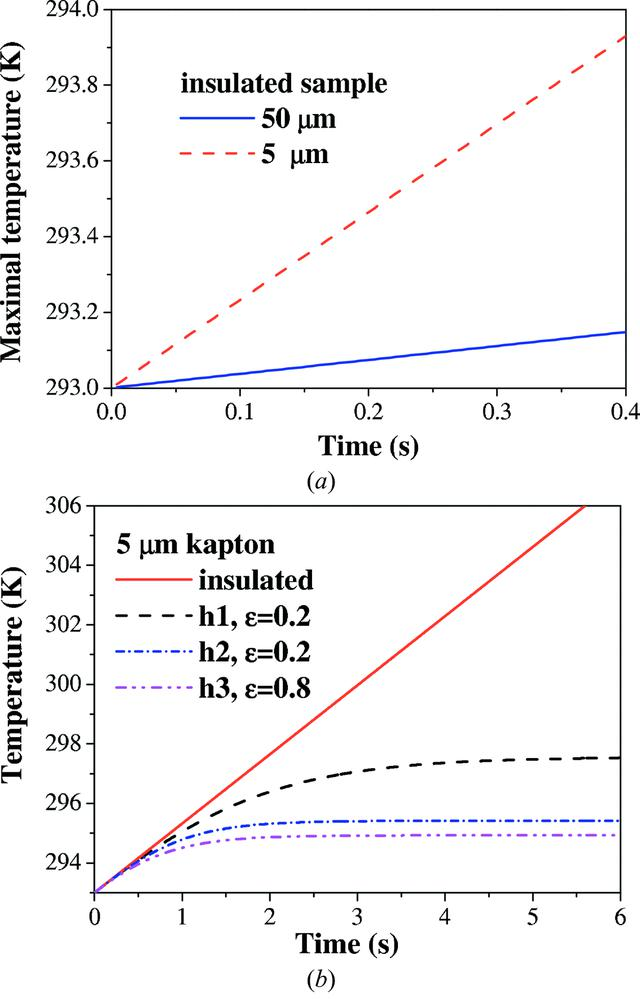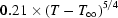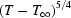disable zoom     view article Figure 6 Results of numerical simulations of 50 nm gold nanospheres dispersed in 1 µm gaps between thick kapton sheets. (a) Maximal temperature growth rate for insulated samples with different thickness of kapton sheets. The dashed line denotes results for 5 µm kapton sheets, the solid line for 50 µm-thick kapton sheets. (b) Maximal temperature growth in the sample with 5 µm kapton sheets for different parameters of heat conduction and emissivity ∊. The solid line denotes the insulated sample, the dashed line denotes conditions with emissivity ∊ = 0.2 and heat conductivity h1 =J m−2 s−1; the dot–dashed line denotes conditions with heat conductivity h2 = 2.1  ×J m−2 s−1 and ∊ = 0.2; the dot–dot–dashed line denotes heat conductivity h3 = h2 as above and emissivity ∊ = 0.8 (i.e. ambient conditions).JOURNAL OFSYNCHROTRONRADIATION
ISSN: 1600-5775
Volume 18| Part 4| July 2011| Pages 580-594
Follow J. Synchrotron Rad.